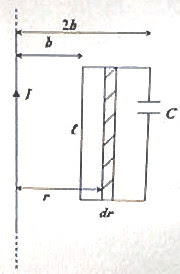## Pages

`“Life is like riding a bicycle.  To keep your balance you must keep moving.”–Albert Einstein`

## Friday, January 1, 2010

### Answer to Free Response Practice Question on Electromagnetism for AP Physics C

“The object of a New Year is not that we should have a new year. It is that we should have a new soul.”

– G. K. Chesterton

Happy New Year…

A free response practice question involving electromagnetism was given to you in the post dated 30th December 2009. As promised, I give below a model answer along with the question:A rectangular wire loop of length and breadth b having negligible resistance is arranged in the plane of an infinitely long straight vertical wire as shown, with the longer sides parallel to the wire. The wire loop contains a capacitor of capacitance C. A steady current ‘I’ flows upwards through the straight wire. Now answer the following questions:

(a) Calculate the magnetic flux through the wire loop.

(b) The loop is rotated through 180º about a central axis (fig.) which is parallel to the straight wire. If the time taken for this rotation is t, determine the magnitude of the emf induced in the loop.

(c) The loop is kept stationary and instead of the steady current I, a current ‘i varying with time t as i = Im sin ωt (where Im and ω are constants) is passed through the straight wire. Calculate the maximum value of the emf induced in the wire loop.

(d) Determine the maximum current induced in the wire loop under the conditions mentioned in part (c) above.

(a) The magnetic field B (produced by the straight current carrying wire) at the loop varies with distance as

B = μ0I/r where ‘r’ is the distance of the point from the straight wire.The magnetic field at the wire loop is directed normally in to the plane of the loop. The magnetic flux through the entire wire loop can be found considering strips parallel to the straight wire. One such strip of width dr at distance r is shown in the figure. This strip has area dA = ℓdr and hence the magnetic flux through this strip is given by

dФ =BdA = 0I/r)ℓdr

The magnetic flux Ф through the entire wire loop is obtained by integrating the above expression between limits r = b and r = 2b.

Therefore, Ф =0Iℓ/2π) b2b(dr/r) = 0Iℓ/2π)[ln(2b) ln(b)]

Or, Ф =0Iℓ/2π) ln(2) = μ0Iℓ ln(2)/

(b) When the wire loop is rotated through 180º, the magnetic flux through the loop changes from Ф to –Ф so that the change of flux is 2Ф. The magnitude of the emf induced in the wire loop is equal to the time rate of change of the magnetic flux through the loop and is equal to 2Ф/t = 2×μ0Iℓ ln(2)/2π∆t = μ0Iℓ ln(2)/π∆t

(c) The magnetic flux linked with the wire loop when a current i = Im sin ωt flows through the straight wire is obtained by replacing I by (Im sin ωt) in the expression for Ф obtained in part (b) above.

Thus Ф = μ0 (Im sin ωt) ln(2)/

The magnitude of the emf induced in the wire loop is equal to dФ/dt.

[The induced emf is – dФ/dt, the negative sign appearing because of Lenz’s law. We ignore the negative sign since we are interested in the magnitude of the emf].

Therefore, induced emf, V = [μ0Imω ln(2) cos ωt]/

The maximum value of induced emf is μ0Imω ln(2) /2π, appropriate to the maximum value of 1 for cos ωt.

(d) The charge Q on the capacitor because of the induced emf is given by

Q = CV = [Cμ0Imω ln(2) cos ωt]/

The induced current iind in the wire loop is given by

iind = dQ/dt = [Cμ0Imω2 ln(2)( sin ωt)]/

The maximum value of induced current is Cμ0Imω2 ln(2) /2π.